# Least Squares, Method of

The following article is from The Great Soviet Encyclopedia (1979). It might be outdated or ideologically biased.

## Least Squares, Method of

a method in the theory of errors for estimating unknown values in terms of the results of measurements containing random errors. The method is also used to approximate a given function by other (simpler) functions and often proves to be useful in the analysis of observational data (calculus of observations).

The method of least squares was proposed by K. Gauss (1794–95) and A. Legendre (1805–06). It was first used in analyzing the results of astronomical and geodetic observations. A rigorous mathematical foundation and a determination of the limits of meaningful applicability of the method of least squares were provided by A. A. Markov (the elder) and A. N. Kolmogorov. The method is now one of the most important branches of mathematical statistics and is widely used for statistical deductions in different branches of science and engineering.

According to Gauss, the basis of the method of least squares is essentially the assumption that the “loss” resulting from the replacement of an exact (unknown) value of a physical quantity μ by its approximate value X, calculated from the results of observations, is proportional to the square of the error: (X − μ)2. Under these conditions, the optimal estimate is naturally assigned a value X that minimizes the mean value of the “loss” and that is free of systematic error. It is precisely this requirement that constitutes the basis of the method of least squares. In the general case, the problem of finding an optimum estimate X in the sense of the method of least squares is extremely complex. So in actual practice the problem is narrowed and X is chosen as the linear function of the observed values, free of systematic errors, that has the minimum mean loss among all linear functions. If the random observation errors obey a normal distribution and if the quantity μ to be estimated depends linearly on the mean values of the observation results (a case often encountered in applications of the method of least squares), then the solution of this problem will simultaneously be the solution of the general problem. The optimum estimate X also obeys a normal distribution with mean value μ and, consequently, the probability density of the random variable X,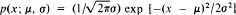attains at x = X a maximum at the point μ = X. (This property expresses the precise meaning of the prevalent assertion in the theory of errors that “the estimate X calculated according to the method of least squares is the most likely value of the unknown parameter μ.”)

Case of a single unknown. Suppose n independent observations giving results Y1, Y2, . . . , Yn have been carried out to estimate the value of the unknown μ, that is, Y1 = μ + δ1, Y2 = μ + δ2, . . . , Yn = μ + δn, where δ1, δ2, . . . , δn are the random errors. (By the definition accepted in classical error theory, random errors are independent random variables with zero mathematical expectation: Eδi = 0; but if Eδi Φ 0, then the Eδi, are said to be systematic errors.) According to the

Table 1
n2345102030
t63.669.925.844.603.252.862.76

method of least squares, we take as the estimate of μ that X for which the following sum of squares is minimized: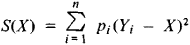where pi = ki2 and σi2 = Dδi = Eδi2 (the coefficient k > 0 may be arbitrarily selected). The quantity pi is called the weight, and σi is called the standard deviation of the measurement with number i. In particular, if the measurements are all equally precise, then σ1 = σ2 = . . . = σn, and in this case we may set p1 = p2 = ... = pn = 1. But if each Y1 is the arithmetic mean of n,i equally precise measurements, then we assume p1 = n1.

The sum S(X) will be least if we select as X the weighted mean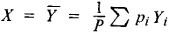where P = Σpi. The estimate ȳ of μ is free of systematic errors and has weight P and variance DY = k/P. In particular, if the measurements are all equally precise, then is the arithmetic mean of the measurement results: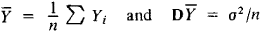It can be proved under certain general assumptions that if the number n of observations is sufficiently large, then the distribution of the estimate ȳ differs little from a normal distribution with mathematical expectation μ and dispersion k/P. In this case, the absolute error of the approximate equality μȳ is less than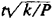with probability nearly equal to the value of the integral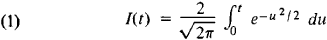For example, I(1.96) = 0.950, I(2.58) = 0.990, and I(3.00) = 0.997.

If the weights pi of the measurements are given and if the factor k remains undetermined prior to the observations, then this factor and the dispersion of the estimate Y can be approximated by the formulas

kS ()/(n1)

and

D = k/ps2 = S ()/[(n – 1)P]

(neither estimate has systematic errors).

In the case of practical importance when the errors δ/ obey a normal distribution, it is possible to find an exact value of the probability with which the absolute error of the approximate equality μ ≈ Ȳproves to be less than ts (t is an arbitrary positive number). This probability, as a function of t, is called the Student distribution function with n − 1 degrees of freedom and is calculated using the formula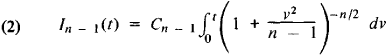where the constant Cn − 1 is selected such that In−1 (∞) =1. For larger n, equation (2) can be replaced by equation (1). However, the use of equation (1) for small n leads to large errors. For example, t = 2.58 corresponds, according to equation (1), to a value of I = 0.99; the true values of t determined for small n as solutions of the corresponding equations In − 1(r) = 0.99 are presented in Table 1.

EXAMPLE. To determine the mass of some body, ten independent equally precise weighings giving results Yi (in grams) are carried out; the results of which are given in Table 2. In the table, Yi is the number of cases for which the weight Yi is observed; n = Σni = 10. Since all the weighings are equally precise, we must set pi = ni and select as the estimate for the unknown weight μ the expression ȳ= ƩniYini = 18.431. For example, setting I9 = 0.95, we find that t = 2.262, using tables of the Student distribution with nine degrees of freedom; therefore, we take the quantity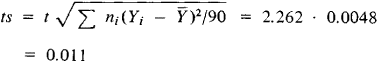as a bound on the absolute error of the approximate equality μ x 18.431. Thus, 18.420 < μ < 18.442.

Case of several unknowns (linear relations). Suppose the n measurement results Y1, Y2, . . . , Yn are related to the m unknowns x1, x2, . . . , xm (m < n) by the independent linear equations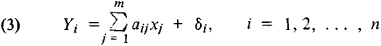where the aij are known coefficients and the δi are independent random errors of the measurements. It is desired to estimate the unknowns xj. (This problem may be considered as a generalization of the preceding one, in which μ = x1 and m = ai1 = 1; i = 1, 2.....n.)

Since Eδi = 0, the mean values of the measurement results yi= EKi are related to the unknowns x1 ,x2, . . . , xm by the linear equations (linear constraints)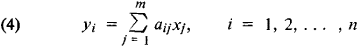Consequently, the unknown values xj constitute a solution of the system (4), whose equations are assumed to be consistent. The precise values of the measured variables yi and the random errors δi are usually unknown, and therefore in place of the systems (3) and (4) it is customary to write what are called conditional equations: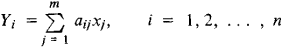According to the method of least squares, we take as the estimates for the unknowns xj. The values Xj for which the sum of the squares of the deviations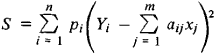will be a minimum (as in the preceding case, pi—the weight of the measurement Yi—is inversely proportional to the dispersion of the random error δi). As a rule, the conditional equations are not consistent—that is, for any values of Xj, the differences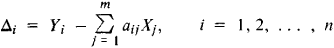in general cannot all vanish, and in this case S = ΣpiΔi2 also cannot vanish. The method of least squares prescribes that we take as the estimates the values of Xj that minimize the sum S. In the unusual cases when the conditional equations are consistent, that is, have a solution, this solution coincides with the estimates obtained by the method of least squares.

The sum of squares S constitutes a quadratic polynomial of the variables Xj. This polynomial attains a minimum at values X1, X2, .... Xm, at which all the first partial derivatives vanish: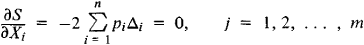Table 2
Yi18.4118.4218.4318.4418.4518.46
ni133111

Therefore, it follows that the estimates Xj obtained by the method of least squares will satisfy a system of “normal” equations, which in the notation proposed by Gauss has the form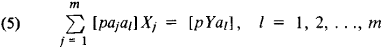where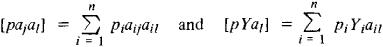The estimates Xj obtained as a result of solving a system of normal equations lack systematic errors (EXj = xj). The variances DXj of the Xj are equal to kdjj/d, where d is the determinant of the system (5) and djj is the minor corresponding to the diagonal element [pajaj]. (In other words, djj/d is the weight of the estimate Xj.) If the proportionality factor k (k is called the variance per unit weight) is unknown, then in order to estimate k, as well as the dispersion D Xj, we use the formulas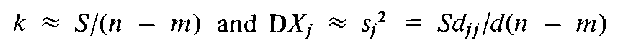(S is the minimum value of the initial sum of squares.) Under certain general assumptions it can be proved that if the number n of observations is sufficiently large, then the absolute error of the approximate equality xiXj is less than tsj with probability close to the value of the integral in equation (1). If the random errors of the observations δi obey a normal distribution, then all the ratios (Xj —xj)/sj are distributed according to Student distribution with n − m degrees of freedom. A precise bound on the absolute error of the approximate equality is carried out here using the integral of equation (2) as in the case of a single unknown. In addition, the minimum value of the sum S is independent, in the probabilistic sense, of X1, X2, . . . , Xm, so that the approximate values of the dispersions of the estimates DXjsj are independent of the estimates Xj themselves.

One of the most typical applications of the method of least squares lies in the “smoothing” observation results Yi taking aij = aj(ti) in equations (3), where aj(t) are known functions of some parameter t (if t is time, then t1, t2, . . . are the moments of time at which the observations are performed). The case of polynomial interpolation, when aj(t) are polynomials [for example, a1(t) = 1, a2(t) = t, a3(t) = t2, . . . ] is often encountered in applications. If t2t1 = t3t2 = ... = tntn − 1 and if the observations are equally precise, tables of orthogonal polynomials can be used to calculate the estimates Xj; such tables are available in many handbooks on modern computational mathematics. A second important case for applications is harmonic interpolation, where trigonometric functions are taken as the aj(t) = cos (j − 1)t, where j = 1, 2, ... , m).

EXAMPLE. To estimate the accuracy of one method of chemical analysis by means of the method of least squares, the concentration of CaO was determined in ten reference samples of previously known composition. The results of the equally precise observations are indicated in Table 3, where 1 is the number of the experiment, ti is the true CaO concentration, Ti is the CaO concentration determined as a result of the chemical analysis, and Yi = Ti, − ti is the error of the chemical analysis.

If the results of the chemical analysis lack systematic errors, then EYi = 0. But if such errors exist, then they may be represented to a first approximation in the form EYi = α + βti, (α is called the systematic error and βti is the method error), or, what is the same thing

E Yi = (α + βt̄) + β(ti - t̄)

where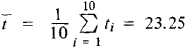To find the estimates α and β, we need only estimate the coefficients x1 = α + βt̄ and x2 = β. In this case, the conditional equations have the form

Yi =x1 + x2(t̄i – t), i = 1, 2,..., 10

Therefore, ai1 = 1 and ai2 = ti all the pi are equal to 1, since we have assumed that the observations are equally precise. Since [a1 a2] = [a2a1] = Ʃ(ti) = 0, the system of normal equations can be written in a particularly simple form: [a1a1]X1 = [Ya1] and [a2a2]X2 = [Ya2] = 1569

where [a1a1] = 10 [a2a2] - Σ(ti – t̄)2 [Ya1] = ΣYi = – 3.5 [Ya2] = ΣYt(tt – t̄) = –8.225

The variances of the components of the solution of this system are given by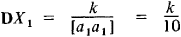and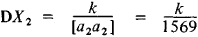where k is the unknown variance per unit weight (in the given case, k is the variance of any of the Yi). Since the components of the solution in this case take the values X1 = −0.35 and X2 = -0.00524, we have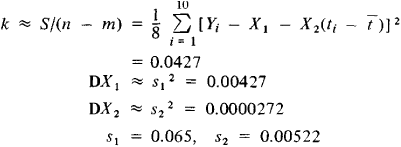If the random observation errors obey a normal distribution, the ratios ǀ Xjxjǀ/ sj (j = 1, 2) are distributed according to the Student distribution. In particular, if the observation results lack systematic errors, then x1 = x2 = 0, and therefore the ratios ǀX1ǀ/s1 and ǀX2ǀ\s2 must obey the Student distribution. Using tables for the Student distribution with n − m = 8 degrees of freedom, we can verify that if in fact x1 = x2 = 0, then each of these ratios will be at most 5.04 with probability 0.999 and at most 2.31 with probability 0.95. In this case, ǀX1ǀ/s1 = 5.38 > 5.04, so that we should reject the hypothesis that systematic errors are absent. At the same time, we must acknowledge that the hypothesis that method errors are absent (x2 = 0) is consistent with the observed results, since ǀX2ǀ/s2 = 1.004 < 2.31. We may therefore conclude that the approximate formula t = T + 0.35 should be used to determine t in terms of the observed value T.

In many cases of practical importance, and particularly in estimating complicated nonlinear relations, the number of unknown parameters may be quite large and the method of least squares will turn out to be effective only when modern computing technology is used.

### REFERENCES

Markov, A. A. Ischislenie veroiatnostei, 4th ed. Moscow, 1924.
Kolmogorov, A. N. “K obosnovaniiu metoda naimen’shikh kvadratov.” Uspekhi matematicheskikh nauk, 1946, vol. 1, issue 1.
Linnik, Iu. V. Metod naimen’shikh kvadratov i osnovy matematiko-statisticheskoi teorii obrabotki nabliudenii, 2nd ed. Moscow, 1962.
Helmert, F. R. Die Ausgleichungsrechnung nach der Methode der kleinsten Quadrate. . ., 2nd ed. Leipzig, 1907.
L. N. BOL’SHEV
Table 3
i12345678910
ti4812.516202531364040
Yi–0.3–0.2–0.4–0.4–0.2–0.5–0.1–0.5–0.6–0.5